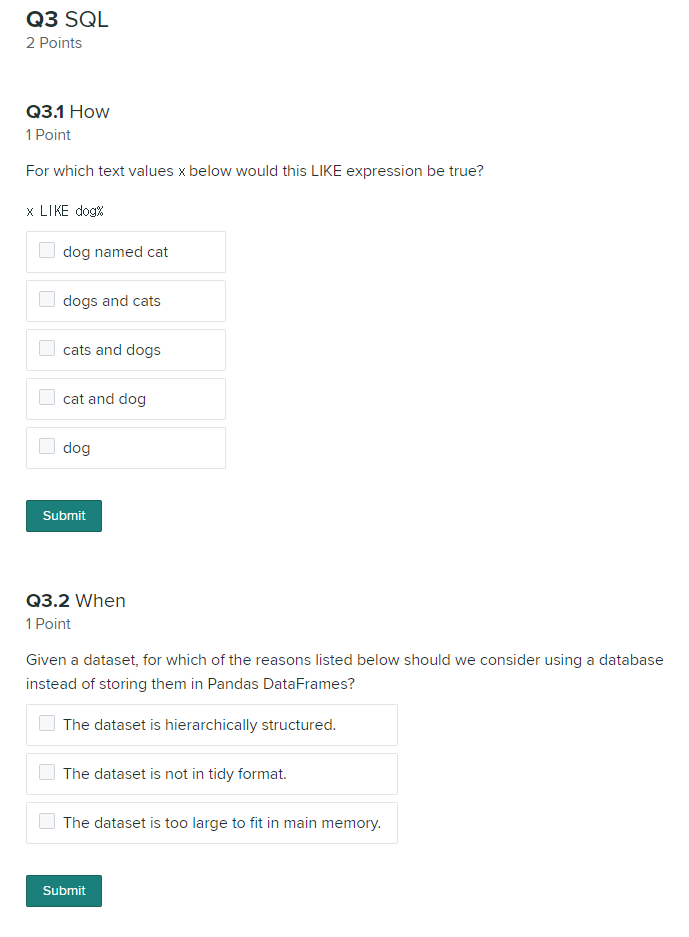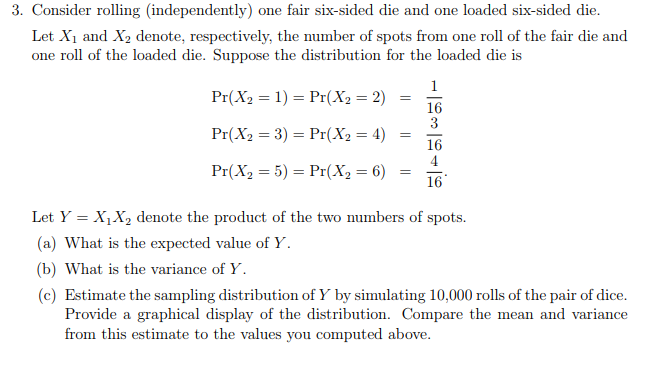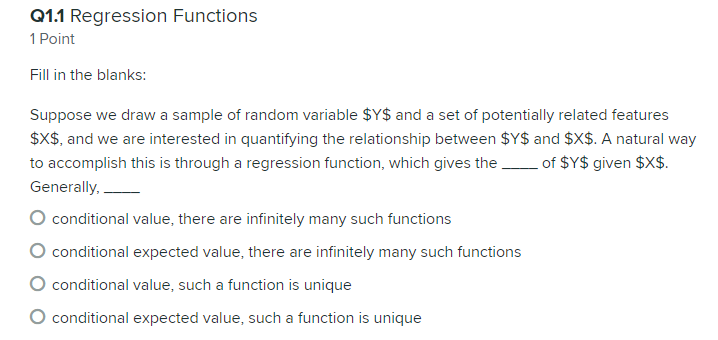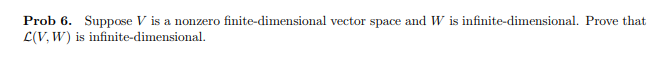Otherwise please don’t answer the question leave it for a capable personal.

There might be more than one answer for the questions.

Q3 SQL 2 Points Q3.1 How Point For which text values x below would this LIKE expression be true? x LIKE dogx dog named cat dogs and cats cats and dogs cat and dog dog Q3.2 When Point Given a dataset, for which of the reasons listed below should we consider using a database instead of storing them in Pandas DataFrames? The dataset is hierarchically structured. The dataset is not in tidy format The dataset is too large to fit in main memory. Submit

Q3.1)

for which text values x below would this like expression is true:

=> following options are correct

=> dog named cat
=>dogs and cats
=>dog

explanation:

like operate is sql is to for searching
it search for particular pattern in column

we have only 2 where conditions with like

one is "%"
and another is "_"

in give question it specifies that dog%

it means it returns all the records where that column value is
starts with dog .

so example: it returns all the names which starts with dog and remaing is what ever.

//------end of question one

Q3.2) Given a dataset for which of the reasons listed below we should consider
database instaad of storing them in Pandas DataFrames?

following are correct

=> the dataset is hierarchically structured
=>the dataset is too large to fit in main memory:

explanation:

first of all Pandas DataFrame is a library avilabe for python.
which is used for fast calculation.
this used of data anylsis.
and it cannot used for persist data.
it performs calculation from sql databases data.
and main thing is it just handles calculation only that fits into data.so it is not at all used for persist data like databases.

#### Earn Coin

Coins can be redeemed for fabulous gifts.

Similar Homework Help QuestionsPlease answer only if your answer is fully sure, otherwise please don’t answer the question leave it for a capable personal. PLEASE!! Don't copy incorrect answers from online. 3. Consider rolling (independently) one fair six-sided die and one loaded six-sided die. Let Xi and X2 denote, respectively, the number of spots from one roll of the fair die and one roll of the loaded die. Suppose the distribution for the loaded die is 16 Pr(X,-5) = Pr(X2 = 6) =...Please find and explain the answer for this question. Answer only if your answer is fully sure, otherwise please don’t answer the question leave it for a capable personal. Please find and explain the answer for this question. Answer only if your answer is fully sure, otherwise please don’t answer the question leave it for a capable personal. Q1.1 Regression Functions 1 Point Fill in the blanks: Suppose we draw a sample of random variable \$Y\$ and a set of...This is an Advanced Linear Algebra Question. Please answer only if your answer is fully sure, Otherwise please don’t answer the question leave it for a capable personal. Please write your writing clearly so it is readable. Prob 6. Suppose V is a nonzero finite-dimensional vector space and W is infinite-dimensional. Prove that L(V. W) is infinite-dimensional.